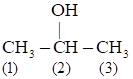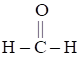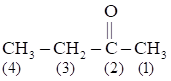### Write answers as directed. Give any four functional groups containing oxygen as the heteroatom in it. Write name and structural formula of one example each.

Question 31.

Write answers as directed.

Give any four functional groups containing oxygen as the heteroatom in it. Write name and structural formula of one example each.

Answer:

Four functional groups are:-

(i) Alcohol

Example: Propan-2-ol(ii) Aldehyde

Example: methanal(iii) Carboxylic acid

Example: Butanoic acid

CH3CH2CH2COOH

(iv) ketones

Examples:- butanone